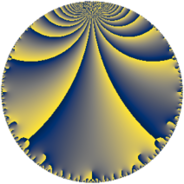# Properties

 Label 43.6.eLevel $43$ Weight $6$ Character orbit 43.e Rep. character $\chi_{43}(4,\cdot)$ Character field $\Q(\zeta_{7})$ Dimension $108$ Newform subspaces $1$ Sturm bound $22$ Trace bound $0$

# Related objects

## Defining parameters

 Level: $$N$$ $$=$$ $$43$$ Weight: $$k$$ $$=$$ $$6$$ Character orbit: $$[\chi]$$ $$=$$ 43.e (of order $$7$$ and degree $$6$$) Character conductor: $$\operatorname{cond}(\chi)$$ $$=$$ $$43$$ Character field: $$\Q(\zeta_{7})$$ Newform subspaces: $$1$$ Sturm bound: $$22$$ Trace bound: $$0$$

## Dimensions

The following table gives the dimensions of various subspaces of $$M_{6}(43, [\chi])$$.

Total New Old
Modular forms 120 120 0
Cusp forms 108 108 0
Eisenstein series 12 12 0

## Trace form

 $$108q - 3q^{2} - 9q^{3} - 331q^{4} + 67q^{5} - 20q^{6} - 624q^{7} + 589q^{8} - 579q^{9} + O(q^{10})$$ $$108q - 3q^{2} - 9q^{3} - 331q^{4} + 67q^{5} - 20q^{6} - 624q^{7} + 589q^{8} - 579q^{9} + 627q^{10} - 220q^{11} - 663q^{12} - 1266q^{13} + 2353q^{14} + 1471q^{15} - 4583q^{16} - 1490q^{17} + 4728q^{18} + 946q^{19} + 14589q^{20} + 776q^{21} + 963q^{22} - 3018q^{23} - 17761q^{24} - 11939q^{25} + 1315q^{26} + 11277q^{27} - 10787q^{28} + 7283q^{29} - 55324q^{30} + 18676q^{31} - 10965q^{32} + 8776q^{33} + 23815q^{34} - 13340q^{35} + 136240q^{36} + 4994q^{37} + 39351q^{38} - 21251q^{39} + 14719q^{40} - 10519q^{41} - 199326q^{42} + 7026q^{43} - 180280q^{44} + 65996q^{45} - 5266q^{46} + 34102q^{47} + 69385q^{48} + 232252q^{49} + 17850q^{50} + 4116q^{51} + 32385q^{52} + 46774q^{53} - 30267q^{54} + 83643q^{55} - 231565q^{56} + 79511q^{57} - 222889q^{58} - 142070q^{59} + 42019q^{60} + 4715q^{61} - 150121q^{62} - 53547q^{63} + 163605q^{64} - 106653q^{65} - 56111q^{66} + 370962q^{67} - 579312q^{68} - 245635q^{69} - 91242q^{70} + 16922q^{71} + 586300q^{72} + 97842q^{73} + 535946q^{74} + 245407q^{75} + 942280q^{76} + 43381q^{77} + 173684q^{78} + 187210q^{79} - 752974q^{80} - 791814q^{81} + 387227q^{82} - 444471q^{83} - 688442q^{84} + 255432q^{85} - 244075q^{86} - 1418850q^{87} + 196837q^{88} + 408972q^{89} - 495568q^{90} - 25764q^{91} + 200120q^{92} + 692536q^{93} + 161449q^{94} + 406105q^{95} + 1096556q^{96} + 266261q^{97} + 527485q^{98} + 212416q^{99} + O(q^{100})$$

## Decomposition of $$S_{6}^{\mathrm{new}}(43, [\chi])$$ into newform subspaces

Label Dim. $$A$$ Field CM Traces $q$-expansion
$$a_2$$ $$a_3$$ $$a_5$$ $$a_7$$
43.6.e.a $$108$$ $$6.897$$ None $$-3$$ $$-9$$ $$67$$ $$-624$$